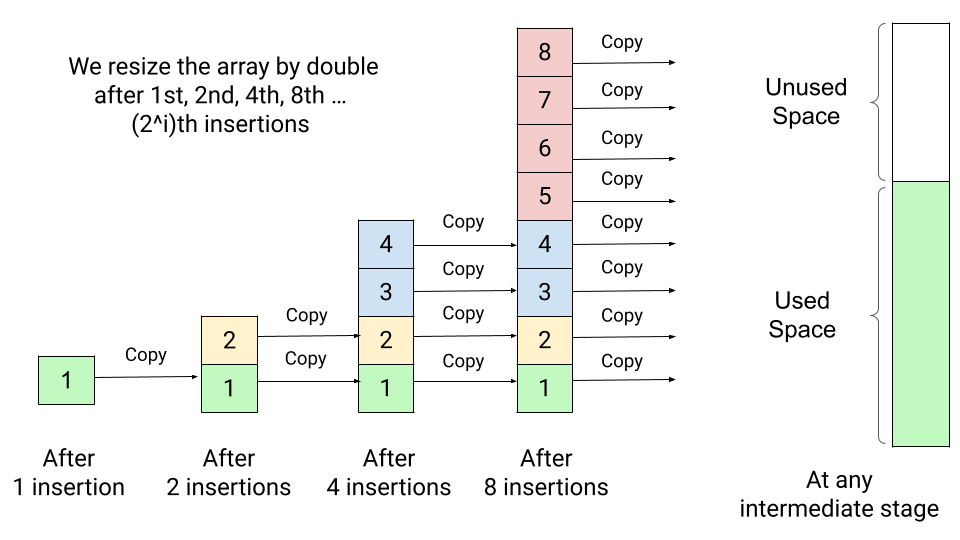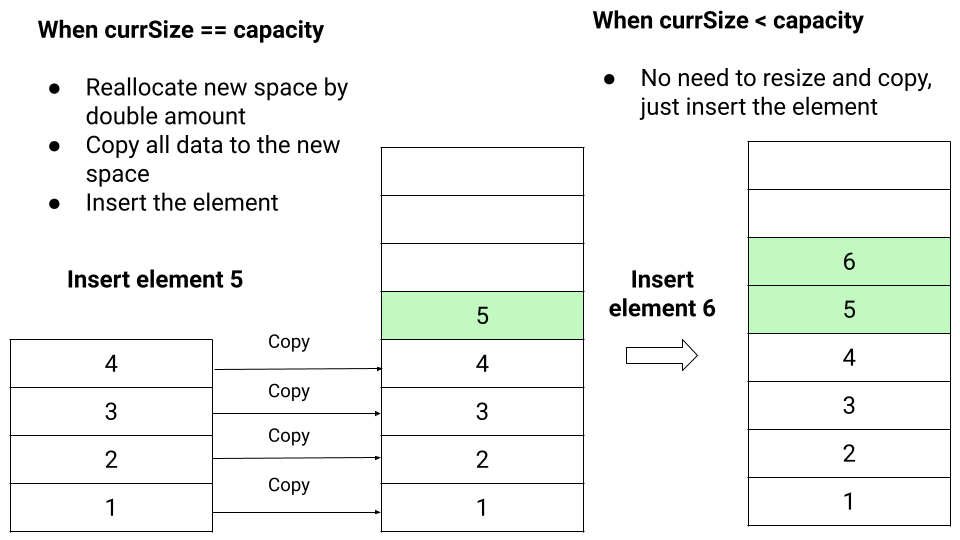# Dynamic Array

## What is Dynamic Array? Why do we use them?

The drawback of arrays is that we cannot adjust their size in the middle of the code execution. Our code will fail to add the (n +1)st element if we have allocated the array size equal to n. We can compensate for this by allocating a huge array, but this could waste a significant amount of space. So what would be an appropriate solution to this problem? Think!

To solve the above problem, we can use the idea of the dynamic array where we can increase the array size dynamically as we need them. Suppose we start with an array size 1 and double its size whenever we run out of space. This process involves allocating a new array of double size, copying the data of the old array to the lower half of the new one, and deleting the space used by the old array. The extra operation involves in this process is recopying the old data on each expansion.

The crucial question is: how many times does an element need to be recopied after n number of insertions? Let's visualize.

• Initially, the array size is 1. So after the first insertion, we need to double the array size to 2 and copy the first element in the new array.
• Now array size is 2, and there is 1 element in the array. So after the second insertion, we need to double the array size to 4 and copy the 2 elements in the new array.
• Similarly, now array size is 4, and there are 2 elements in the array, so no need to resize the array after the 3rd insertion. But after the 4th insertion, the array gets full, and we need to double the array size to 8 and copy the 4 elements in the new array.

This process of doubling continues if we insert 8th, 16th, 32nd ... elements. In general, after i number of doubling, the array size would be 2^i, and there are 2^(i-1) elements in the array. So there is no need to resize the array after 2^(i-1) + 1 to 2^i - 1 insertion. But after the 2^i th insertion, the array gets full, and we need to double the array size to 2^(i + 1) and copy the 2^i elements in the new array.### Amortized analysis of insertion in Dynamic Array

From the above observations, after i number of doubling, if there are n elements in the array, then 2^i = n => i = logn. So for n insertion operations, we double the array size logn times.

Total number of copy operations after logn number of doubling

= 1 + 2 + 4 + ..... n/4 + n/2 + n

= n + n/2 + n/4 + ..... + 4 + 2 + 1

= n (1 + 1/2 + 1/4 + ..... + 4/n + 2/n + 1/n)

~ n (1 + 1/2 + 1/4 + ..... + 4/n + 2/n + 1/n + .... till infinity)

~ n/(1 - 1/2) ~ 2n

Note: In the above calcualtion, we have used the idea of infinite geometric series => 1 + 1/r + 1/r^2 + 1/r^4 + ...till infinity = 1/(1 - r)

• The total number of copy operations after n number of insertion = 2n
• Total copy operation per insertion = 2n/n = 2 = O(1)
• Thus, each of the n elements moves only two times on average.

The primary thing lost using dynamic arrays is guaranteeing that each array access takes constant time in the worst case. Now all the queries will be fast, except for few queries which trigger array doubling. One idea is clear: expanding the array size by a constant factor 2 ensures that inserting n elements takes O(n) time overall, meaning that each insertion takes amortized constant time.

## Dynamic array operations

For the implementation of the dynamic array in C or C++ or Java or some other programming language, let's define three things:

• A: pointer to the data array
• currSize: current number of elements in the dynamic array A[]. It is initialized with the value 0 at the start.
• capacity: total number of elements that a dynamic array A[] can hold before it must resize. It is initialized with the value 1 at the start.

### Resizing the array capacity by double: reSizeArray()

This is required when after some sequence of insertion, allocated array space gets full with the defined capacity. So we perform this operation when currSize == capacity during the insertion.

• We double the value of array capacity and declare a new array temp[] of size 2*capacity.
• Now we run a loop from i = 0 to capacity - 1 and copy all the elements from the original array A[] to the new array temp[].
• We free the space used by A[] and initialize it with the new array temp[].
• Finally, we double the value of the capacity.
``````void reSizeArray()
{
if (currSize == capacity)
{
int temp[2 * capacity]
for (int i = 0; i < capacity; i = i + 1)
temp[i] = A[i]
free(A)
A = temp
capacity = 2 * capacity
}
}
``````

### Insertion at the end: insertAtEnd(int x)

• If(currSize == capacity), then we resize the array i.e. reallocate new space and copy all data to the new space. Doing all that takes O(n) time, where n is the number of elements in our array.
• Then we add an element x at the end.``````void insertAtEnd(int x)
{
if (currSize == capacity)
resizeArray()
A[currSize] = x
currSize = currSize + 1
}
``````

### Insertion at the middle: insertAtMiddle(int index, int x)

• If(currSize == capacity), then we resize the array by double.
• Now we move elements from index to currSize - 1 to one index upward. This process will create a space for the newly arriving element x.
• Now we insert the element x at A[index] and increase currSize by 1.
``````void insertAtMiddle(int index, int x)
{
if (currSize == capacity)
reSizeArray()

for (int i = currSize - 1; i > index; i = i - 1)
A[i] = A[i - 1]

A[index] = x
currSize = currSize + 1
}
``````

### Resizing the array capacity by half: shrinkArray()

After some sequence of deletion, the currSize of the array may be significantly less in comparison to the current capacity. So dynamic array can also deallocate some of the space if its size drops below a certain threshold, such as 1/4th of the current capacity. This would help in reducing the wastage of extra space.

We perform this operation when currSize == capacity/4 during the insertion.

• We declare a new array temp[] of size capacity/2.
• Now we run a loop from i = 0 to currSize -1 and copy all the elements from the original array A[] to the temp[] array.
• We update the new capacity equal to capacity/2 and free the space used by A[].
• Now we initialize A[] with the new array temp[].
``````void shrinkArray()
{
if (currSize == capacity/4)
{
int temp[capacity/2]
for (int i = 0; i < currSize; i = i + 1)
temp[i] = A[i]
capacity = capacity/2
free(A)
A = temp
}
}
``````

### Deletion at the end: deleteAtEnd()

We need to check the current size of the array. if(currSize == 0), then array is empty or there will no element in the array. if(currSize > 0), then we remove the element at index currSize - 1 and reduce currSize by 1.

``````void deleteAtEnd()
{
if (currSize == 0)
print("dynamic array is empty!")
else
{
A[currSize - 1] = INT_MIN
currSize = currSize - 1
if (currSize == capacity/4)
shrinkArray()
}
}
``````

### Deletion at the middle: deleteAtMiddle(int index)

• We need to check the current size of the array. if(currSize == 0), array is empty or there will no element in the array. Otherwise, we move all the elements from currSize - 1 to index by 1 downward.
• Now we set value at A[currSize - 1] equal to 0 and decrement currSize by 1.
``````void deleteAtMiddle(int index)
{
if (currSize == 0)
print("dynamic array is empty!")
else
{
for (int i = index; i < currSize - 1; i = i + 1)
A[i] = A[i + 1]

A[currSize - 1] = INT_MIN
currSize = currSize - 1
if (currSize == capacity/4)
shrinkArray()
}

}
``````

### Class-based pseudocode implementation of the dynamic array

``````public class DynamicArray
{
int A[]
int currSize
int capacity

public:
DynamicArray()
{
A = new int
currSize = 0
capacity = 1
}

void insertAtEnd(int x)
{
if (currSize == capacity)
resizeArray()
A[currSize] = x
currSize = currSize + 1
}

void reSizeArray()
{
if (currSize == capacity)
{
int temp[2 * capacity]
for (int i = 0; i < capacity; i = i + 1)
temp[i] = A[i]
free(A)
A = temp
capacity = 2 * capacity
}
}

void shrinkArray()
{
if (currSize == capacity/4)
{
int temp[capacity/2]
for(int i = 0; i < currSize; i = i + 1)
temp[i] = A[i]
capacity = capacity/2
free(A)
A = temp
}
}

void insertAtMiddle(int index, int x)
{
if (currSize == capacity)
reSizeArray()

for (int i = currSize - 1; i > index; i = i - 1)
A[i] = A[i - 1]

A[index] = x
currSize = currSize + 1
}

void deleteAtEnd()
{
if (currSize == 0)
print("dynamic array is empty!")
else
{
A[currSize - 1] = INT_MIN
currSize = currSize - 1
if (currSize == capacity/4)
shrinkArray()
}
}

void deleteAtMiddle(int index)
{
if (currSize == 0)
print("dynamic array is empty!")
else
{
for (int i = index; i < currSize - 1; i = i + 1)
A[i] = A[i + 1]

A[currSize - 1] = INT_MIN
currSize = currSize - 1
if (currSize == capacity/4)
shrinkArray()
}
}
}
``````

### Critical concepts to think in dynamic array!

• Analyze and compare the time complexities of the above dynamic array operations with the normal array.

Dynamic array is supplied with standard libraries in many modern programming languages. In C++, it is called a vector, and in Java, it has two implementations: ArrayList and Vector.

• The growth factor for the dynamic array depends on several things like a time-memory trade-off, algorithms used inside the memory allocator, etc.
• For growth factor k, the average time per insertion operation is about k/(k−1), where the maximum number of wasted space will be (k−1)n.
• For the simplicity of the analysis, we have used the growth factor k = 2. But if the memory allocator uses a first-fit allocation algorithm, then growth factor values such as k = 2 can cause dynamic array expansion to run out of memory even though a significant amount of memory may still be available.
• There have been several discussions on the ideal values of the growth factor. Some experts suggest the golden ratio value as a growth factor, but library implementation of different programming languages uses different values. Explore and think!

Enjoy learning, Enjoy algorithms!

Get well-designed application and interview centirc content on ds-algorithms, machine learning, system design and oops. Content will be delivered weekly.